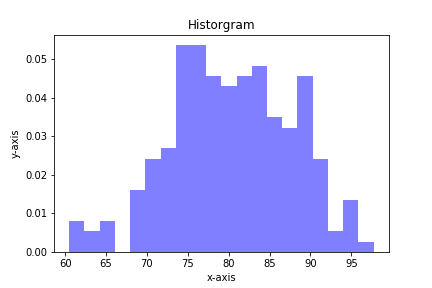## Estimating the Deflection of a Truncated Cone using PythonIn this post, we will complete a problem that might come up in a Strength of Materials class. We'll estimate the deflection of a truncated cone given an applied load using Python.

### The Problem¶

Below is an engineering mechanics problem that can be solved in Python. Follow along this post …

## Plotting Bond Energy vs. Distance with Python and MatplotlibAtoms in solid materials like steel and aluminum are held together with chemical bonds. Atoms of solid materials are more stable when they are chemically bonded together, and it takes energy to separate atoms which are joined together with a chemical bond. The bonding energy associated with a chemical bond …

## Hear my story about moving from MATLAB to Python on TalkPython

I was a guest on the TalkPython podcast with Michael Kennedy! We talked about teaching Python at a Community College and how I moved a college engineering class from MATLAB to Python using JupyterHub.

## Quiver plots using Python, matplotlib and Jupyter notebooksA quiver plot is a type of 2D plot that shows vector lines as arrows. Quiver plots are useful in electrical engineering to visualize electrical potential and valuable in mechanical engineering to show stress gradients. In this post, we will build three quiver plots using Python, matplotlib, numpy, and Jupyter notebooks.

## Plotting Histograms with matplotlib and PythonHistograms are a useful type of statistics plot for engineers. A histogram is a type of bar plot that shows the frequency or number of values compared to a set of value ranges. Histogram plots can be created with Python and the plotting package matplotlib. The plt.hist() function creates …